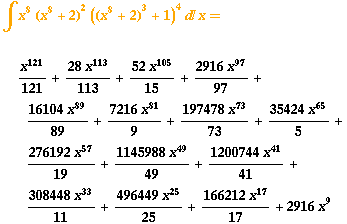SEARCH HOMEMath Central Quandaries & QueriesSubject: integrals (calculus) Name: James Who are you: Student how do you integrate: 1) x^8 (x^8 + 2)^2 ((x^8 + 2)^3 + 1)^4 i've tried using u = x^8 + 2 2) (X^3) / (sqrt(x^2 -1)) 3)1 / ((x^1/2) + (X^1/4)) 4) (tanx^4) (tanx^2)^2 (secx^2 -1)^2 NOW WHAT? THANK YOUJames,

I don't see anything to do here except to multiply out the expression, get a polynomial of order 120 and then integrate. I used The Integrator that uses Mathematica and it seems like that is the technique that Mathematica used. Here is the output I received from The IntegratorPennyMath Central is supported by the University of Regina and The Pacific Institute for the Mathematical Sciences.